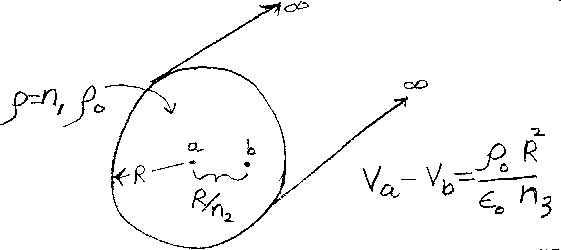Problem B12: Consider the infinitely long solid cylinder shown in the figure above. The cylinder has a radius R, and has a uniform charge volume density of n1 ρ0 > 0. What is the voltage difference between a point located a distance of r = R/n2, labeled "b", and the center of the cylinder, labeled "a"? That is if V(a)-V(b) = ρ0R2/(ε0 n3), what is n3 ?n1 = n2 = Input n3:
If you are currently in my class, you can record your grade by entering your name and student ID number (without the leading zeros) below and clicking on "record grade".
 First Name = Last Name = ID = Problem: